## Info

Publication number
JP3683733B2
JP3683733B2 JP4173399A JP4173399A JP3683733B2 JP 3683733 B2 JP3683733 B2 JP 3683733B2 JP 4173399 A JP4173399 A JP 4173399A JP 4173399 A JP4173399 A JP 4173399A JP 3683733 B2 JP3683733 B2 JP 3683733B2
Authority
JP
Japan
Prior art keywords
signal
frequency offset
estimated
plurality
Prior art date
Legal status (The legal status is an assumption and is not a legal conclusion. Google has not performed a legal analysis and makes no representation as to the accuracy of the status listed.)
Expired - Fee Related
Application number
JP4173399A
Other languages
Japanese (ja)
Other versions
JP2000244454A (en
Inventor

Original Assignee

Priority date (The priority date is an assumption and is not a legal conclusion. Google has not performed a legal analysis and makes no representation as to the accuracy of the date listed.)
Filing date
Publication date
Application filed by 三菱電機株式会社 filed Critical 三菱電機株式会社
Priority to JP4173399A priority Critical patent/JP3683733B2/en
Publication of JP2000244454A publication Critical patent/JP2000244454A/en
Application granted granted Critical
Publication of JP3683733B2 publication Critical patent/JP3683733B2/en
Anticipated expiration legal-status Critical
Application status is Expired - Fee Related legal-status Critical

## Images

•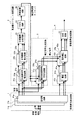•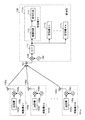•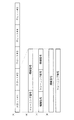•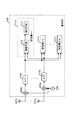•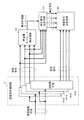•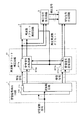•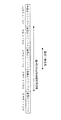••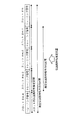•## Description


BACKGROUND OF THE INVENTION
The present invention relates to a code division multiple access (CDMA) receiver in the field of wireless communication such as a car phone and a mobile phone, and in particular, communication with good quality by correcting a phase shift of a received signal. It is related with the CDMA receiver which implement | achieves.

[Prior art]
A conventional CDMA receiver will be described below. First, an outline of a communication system using CDMA will be described. For example, each of N mobile units accommodated in one base station generates a transmission spread signal spread with an individual spread code by an internal transmitter, and further transmits a transmission spread signal based on a frequency generated by an oscillator. Up-convert and transmit the signal via the antenna. The transmission waves transmitted from N mobile devices are received by the base station via the antenna as multiplexed reception waves.

The received wave is down-converted based on the frequency generated by the oscillator, and converted into a digital received spread signal by an analog / digital (A / D) converter inside the base station. The reception spread signal output from the A / D converter is demodulated and decoded by a receiver corresponding to each user (mobile device).

References relating to the CDMA receiver used in the above-described communication system using CDMA include, for example, “High-accuracy channel estimation method using a plurality of pilot blocks in DS-CDMA” by Ando and Sawahashi (The IEICE Tech. RCS 96-72). , PP.45-50 (1996-8)).

The receiver demodulation circuit described in “High-Accuracy Channel Estimation Method Using Plural Pilot Blocks in DS-CDMA” will be described below. FIG. 10 shows a configuration of a demodulation circuit in a conventional CDMA receiver. Note that the transmission signal transmitted by the mobile device is configured such that the information signal and the training signal are spread with different spreading codes, and code division multiplexed and transmitted (see FIG. 3D). In FIG. 10, 71 is a reception signal extraction circuit, 72 is a correction signal generation circuit, and 73 is a reception signal synthesis circuit.

The despreading circuits 711a, 711b,... 711c in the reception signal extraction circuit 71 perform despreading by a spreading code corresponding to the reception signal extracted from the reception spread signal by known means. At this time, the same received signal is extracted for each component that can be temporally divided (hereinafter, each component is referred to as a path). For the signal as shown in FIG. 3D, the training signal and the information signal are separated and extracted. Further, there are as many despreading circuits as the number of J paths.

The transmission path characteristic estimation circuits 721a, 721b,... 721c (J) in the correction signal generation circuit 72 obtain the estimated transmission path characteristics h (m, j) corresponding to the mth slot by the processing of equation (1). Calculate (j = 1,..., J).
h (m, j) = Σr T (N, m, j) × I (n, m) * (1)
R T (N, m, j) is the nth symbol of the training signal portion of the jth path of the mth slot in the received signal. I (n, m) is the nth symbol of the transmitted training signal in the mth slot. And a * Is the complex conjugate of a. In addition, Σ in equation (1) is n = 1,..., N T The sum of N T Is the number of symbols of the training signal in the slot.

The estimated transmission line characteristic averaging circuits 722a, 722b,... 722c average the estimated transmission line characteristic of each path. For example, in the estimated transmission path characteristic averaging circuit 722a, the estimated transmission path characteristics h (mi, 1) of the past four slots are stored by the shift register 723 (i = 1,..., 4), and the current estimation is performed. Each estimated transmission path characteristic is output to the multipliers 724 to 728 together with the transmission path characteristic h (m, 1). Multipliers 724 to 728 weight estimated transmission path characteristics h (mi, 1) based on the fixed weight coefficient output from weight coefficient generation circuit 729. Then, the summation circuit 730 calculates the summation of the outputs of the multipliers 724 to 728 and outputs H (m−2, 1) as a correction signal to the signal correction circuit 732a. The same operation is performed for other paths.

The delay circuits 731a, 731b,... 731c in the reception signal synthesis circuit 73 delay and output the information signal of the reception signal for a time required for generating the correction signal.

The signal correction circuits 732a, 732b,... 732c are information signals r of received signals output from the delay circuits 731a, 731b,. D (N, m-2, j) is corrected by the correction signal H (m-2, j) (n = 1,..., N D , J = 1,..., J). Where N D Is the number of symbols of the information signal in the slot, and the information signal r D (N, m-2, j) is corrected by equation (2).
r D '(N, m-2, j) = r D (N, m-2, j) x H (m-2, j) * (2)
R D '(N, m-2, j) is a corrected received signal.

The combining circuit 733 generates a corrected received signal r D The sum of '(n, m-2, j) is calculated, and the soft decision value of the demodulation result is output as reception information.

[Problems to be solved by the invention]
However, in the above-described conventional CDMA receiver, when transmission signals from a plurality of mobile devices multiplexed by codes are received, each transmission signal has an individual frequency offset due to variations in the absolute accuracy of the oscillator in each mobile device. (Frequency shift). In this case, the estimated transmission path characteristics whose phase is shifted due to the frequency offset are averaged. Therefore, in the conventional CDMA receiver, in order to reduce the influence due to the phase shift, the number of slots to be averaged is reduced, or the weight coefficient of the slots that are separated in time is reduced.

As described above, in the conventional CDMA receiver, it is necessary to reduce the number of slots to be averaged or to reduce the weighting factor of slots that are separated in time, and accordingly, the accuracy of the correction signal is deteriorated. As a result, there is a problem that the quality of the received signal (decoded data) is deteriorated.

Accordingly, the present invention has been made in view of the above, and after correcting the phase shift of the estimated transmission path characteristic before averaging, the correction signal is further considered in consideration of the phase shift of the received signal. An object of the present invention is to obtain a CDMA receiver that realizes high-quality communication.

[Means for Solving the Problems]

According to the present invention, despreading is performed with a spreading code corresponding to each user, a plurality of reception signals having different arrival times are extracted, frequency offsets of the plurality of reception signals are estimated, and the plurality of reception signals Then, a correction signal corresponding to each received signal is generated from the estimated frequency offset, and the received signal corresponding to each path after being corrected by the highly accurate correction signal is synthesized. As a result, the present invention can output a soft decision value or a decision value with high accuracy, and at the same time, can reduce the error rate of data after error correction by a subsequent decoder, resulting in quality. Good communication.

The CDMA receiver according to the next invention is characterized by receiving a transmission spread signal from a single user.

According to the present invention, even when receiving a transmission spread signal from a single user, it is possible to output a soft decision value or a decision value with high accuracy, and accompanying this, data after error correction by a later stage decoder Error rate can be reduced, and as a result, high-quality communication can be performed.

In the CDMA receiver according to the next invention, the frequency offset estimation means calculates transmission path characteristics for calculating estimated transmission path characteristics corresponding to each received signal based on a plurality of received signals extracted by the received signal extraction means. Based on the estimation means (corresponding to transmission path characteristic estimation circuits 21a, 21b,... 21c in the embodiments described later) and the estimated transmission path characteristics corresponding to each received signal, the estimated phase deviation corresponding to each received signal is calculated. A phase deviation estimation circuit (corresponding to phase deviation estimation circuits 22a, 22b,... 22c) to be calculated, and phase deviation synthesis means (phase deviation synthesis) for synthesizing estimated phase deviations corresponding to the received signals and calculating a synthesized phase deviation. And an averaging means (equivalent to the averaging circuit 24) for calculating the estimated frequency offset by averaging the combined phase deviation. That.

According to the present invention, before averaging, correction of the phase shift of the received signal or correction of the phase shift of the estimated transmission path characteristic calculated for a short section is performed, and then the estimated transmission path characteristic is averaged. Do. As a result, it is possible to lengthen the time for averaging the estimated transmission path characteristics, and it is possible to generate a correction signal with higher accuracy.

In the CDMA receiver according to the next invention, the correction signal generating means changes the length of the received signal used for calculation of the estimated transmission path characteristic according to the state of the transmission path.

According to the present invention, when the transmission path characteristics fluctuate gently, the averaging time of the estimated transmission path characteristics is set to a range in which it can be assumed that there is almost no fluctuation of the transmission path characteristics. On the other hand, when the transmission path characteristics fluctuate quickly, the time for averaging the estimated transmission path characteristics is shortened. In this way, a highly accurate correction signal that does not depend on the fluctuation amount of the transmission path characteristic is calculated by changing the time for averaging the estimated transmission path characteristic according to the fluctuation amount (speed of fluctuation) of the transmission path characteristic. Can do.

In the CDMA receiver according to the next invention, transmission spread signals of a plurality of users spread and multiplexed by the individual spreading codes are transmitted to a plurality of antennas (corresponding to antennas 207a and 207b in the embodiments described later). The reception signal extraction unit, the frequency offset estimation unit, the correction signal generation unit, and the reception signal synthesis unit demodulate a plurality of reception signals having different arrival times.

According to the present invention, transmission spread signals of a plurality of users spread and multiplexed with individual spreading codes are diversity-received by a plurality of antennas, so that higher quality communication can be performed.

The CDMA receiver according to the next invention is further characterized in that a transmission spread signal from a single user is received by a plurality of antennas.

According to the present invention, even when a transmission spread signal from a single user is received, since diversity reception is performed by a plurality of antennas, higher quality communication can be performed.

The CDMA receiver according to the next invention further includes frequency offset correction means for correcting the reception signal output from the reception signal extraction means based on the estimated frequency offset estimated by the frequency offset estimation means. It is characterized by.

According to the present invention, since the frequency offset is corrected for the received signal, it is not necessary to correct the frequency offset in generating the correction signal.

DETAILED DESCRIPTION OF THE INVENTION
Embodiments of a CDMA receiver according to the present invention will be described below in detail with reference to the drawings. Note that the present invention is not limited to the embodiments. Further, the present invention can be used for reception on both the base station and the mobile station side.

Embodiment 1 FIG.
FIG. 1 is a diagram showing a configuration of a demodulation circuit of a CDMA receiver according to the present invention. First, the positioning of a CDMA receiver in a CDMA (Code Division Multiple Access) communication system will be described. FIG. 2 is a diagram showing an outline of a communication system using CDMA.

In FIG. 2, 101a, 101b,... 101c are a plurality of mobile devices, and 106 is a base station that accommodates these mobile devices. Also, in mobile devices 101a, 101b,... 101c, 102a, 102b,... 102c are transmitters that spread transmission signals with individual spreading codes, and 103a, 103b,... 103c are oscillators that generate transmission frequencies. 104a, 104b,... 104c are mixers for up-converting the transmission spread signal, and 105a, 105b,.

In the base station 106, 107 is an antenna, 108 is an oscillator, 109 is a mixer that down-converts the received spread signal, 110 is an A / D converter that converts the received spread signal into a digital received spread signal, 111a, 111b,... 111c are receivers that demodulate and decode received signals by the internal demodulation circuit 112 and decoding circuit.

Here, the operation of each configuration described above in the CDMA communication system will be briefly described. For example, N mobile devices 101a, 101b,... 101c generate transmission spread signals spread by individual spreading codes at transmitters 102a, 102b,. Then, based on the frequency generated by the corresponding oscillator 103a, 103b,... 103c, the transmission spread signal is up-converted by the mixers 104a, 104b,. A signal is transmitted from each mobile device.

The transmission waves transmitted from the N mobile devices 101a, 101b,... 101c are received by the base station 106 via the antenna 107 as multiplexed reception waves. The received wave is down-converted by the mixer 109 based on the frequency generated by the oscillator 108 and converted into a digital received spread signal by an A / D (analog / digital) converter 110. The reception spread signal output from A / D converter 110 is demodulated and decoded by demodulation circuit 112 and decoding circuit 113 at receivers 111a, 111b,... 111c corresponding to each user (mobile device).

In the CDMA communication system configured as described above, the configuration of the CDMA receiver of the present invention shown in FIG. 1 is positioned as a portion corresponding to the demodulation circuit 112. A specific example of a transmission signal transmitted by each mobile device is shown in FIG. For example, in the illustrated transmission signal, as shown in FIG. 3A, a block of signals called slots is continuously formed. In FIGS. 3B, 3C, and 3D, the configuration of each slot is shown. An example is shown.

Specifically, FIG. 3B shows a configuration in which a training signal known on the receiver side is preceded by an information signal (an encoded signal, including a control signal). is there. FIG. 3C shows a configuration in which there is a training signal known on the receiver side in the middle of an information signal (encoded signal), and a configuration in which there is a training signal at the end is also conceivable. . FIG. 3D shows a configuration in which the information signal and the training signal are spread with different spreading codes, and are transmitted by code division multiplexing. In addition, it is conceivable that the training signal portion of FIG. 3D has the configuration of FIGS. 3B and 3C, but for the sake of convenience of explanation, the configuration of FIG. To do.

Hereinafter, the demodulation circuit of the CDMA receiver according to the present invention will be described in detail. In FIG. 1, 1 is a received signal extracting circuit, 2 is a frequency offset estimating circuit, 3 is a correction signal generating circuit, and 4 is a received signal synthesizing circuit. In the received signal extraction circuit 1, reference numerals 11a, 11b,... 11c are despreading circuits.

In the frequency offset estimation circuit 2, 21a, 21b, ... 21c are transmission path characteristic estimation circuits, 22a, 22b, ... 22c are phase deviation estimation circuits, 23 is a phase deviation synthesis circuit, and 24 is an average. 25 is an angle conversion circuit. In the correction signal generation circuit 3, 31a, 31b,... 31c are estimated transmission line characteristic averaging circuits. In the received signal synthesis circuit 4, 41a, 41b,... 41c are delay circuits, 42a, 42b,... 42c are signal correction circuits, and 43 is a synthesis circuit.


Next, the frequency offset estimation circuit 2 calculates an estimated frequency offset based on the received signal extracted by the received signal extraction means 1. The operation of the frequency offset estimation circuit 2 is as follows. For example, in the transmission line characteristic estimation circuits 21a, 21b,... 21c, the estimated transmission line characteristic h (m, j) corresponding to the mth slot is calculated (j = 1,. , J).
h (m, j) = Σr T (N, m, j) × I (n, m) * (3)
R T (N, m, j) is the nth symbol of the training signal portion of the jth path of the mth slot in the received signal. I (n, m) is the nth symbol of the transmitted training signal in the mth slot. And a * Is the complex conjugate of a. Also, Σ in equation (3) is n = 1,..., N T Is the sum of N and N here T Indicates the number of symbols of the training signal in the slot.

In the phase deviation estimation circuits 22a, 22b,... 22c, as shown in the equation (4), the current estimated transmission path characteristic h (m, j) and the estimated transmission path characteristic h (m−1, j in the past of one slot). ) And the complex conjugate of each, and the result is output as the complex phase deviation θ (m, j).
θ (m, j) = h (m, j) × h (m−1, j) * (4)

The phase deviation synthesizing circuit 23 calculates the sum of the complex phase deviations of each path as shown in the equation (5), and outputs φ (m) as the synthesized phase deviation.
φ (m) = Σθ (m, j) (5)
In the equation (5), Σ represents the sum in j = 1,.

The averaging circuit 24 calculates the time average of the combined phase deviation φ (m) and outputs the result as an estimated complex frequency offset. For example, as a method for realizing averaging, there is the following equation.
F (m) = Σφ (m + 1−i) (6)
In the equation (6), Σ is i = 1,..., N AVE Is the sum of N and N here AVE Indicates the number of samples to be averaged. Further, as other averaging realizing means by the averaging circuit 24, for example, a change as shown in Expression (7) is also possible.

F (m) = (1-δ) × F (m−1) + δ × φ (m) (7)
Note that δ is a parameter for determining the averaging time constant, and takes a value smaller than 1. Further, the averaging means is not limited to this, and other methods may be applied.

The angle conversion circuit 25 converts the estimated complex frequency offset into an angle as shown in Expression (8), and outputs the result as an estimated frequency offset.
f (m) = arctan (F (m)) (8)

Next, the correction signal generation circuit 3 calculates a correction signal for the reception signal based on the reception signal extracted by the reception signal extraction circuit 1 and the estimated frequency offset estimated by the frequency offset estimation circuit 2. To do. The operation of the correction signal generation circuit 3 is as follows.

For example, the estimated transmission line characteristic averaging circuits 31a, 31b,... 31c each input a received signal corresponding to each path, and calculate the estimated transmission line characteristic while correcting the phase rotation due to the frequency offset of the received signal. FIG. 7 shows an operation example when the (m-2) th to mth slots are used for averaging the estimated transmission path characteristics, and the result is used in the (m-1) th slot. It is. This process is shown in Equation (9).
The inner Σ is n = 1,..., N T , And Σ on the outer side represents the sum of i = 0,. SQRT (-1) is a route (complex number) of -1. Accordingly, the signal correction section shown in FIG. 7 can be corrected using the estimated transmission path characteristic h ′ (m−1, j) as a correction signal.

Further, by performing the processing of Expression (10), the estimated transmission path characteristic h ′ (m−1, j) obtained by correcting the frequency offset for each symbol can be used as the correction signal.

Further, as shown in FIG. 8, the amount of fluctuation (for example, Doppler frequency) of the transmission path is estimated by a known method, and the length of the slot used for averaging the estimated transmission path characteristics is switched according to the value. Is also possible.

Further, as shown in FIG. 9, the estimated transmission path characteristic h (m−1, j) corresponding to each slot estimated in the transmission path characteristic estimation circuits 21a, 21b,. Regardless of the processing, the estimated transmission path characteristics can be averaged by the processing of Expression (11).
Here, Σ indicates the sum total at i = 0,. INT (a) is a value obtained by rounding down the decimal point of a, and N slot Is the number of slots for averaging the estimated transmission path characteristics. Also, N according to the fluctuation amount of the transmission path slot It is also possible to change.

Furthermore, the estimated transmission path characteristic h ′ (m−INT (N slot / 2), j) obtained by correcting the frequency offset for each symbol can be used as a correction signal.

Finally, the received signal synthesis circuit 4 performs phase compensation on the received signal corresponding to each path by the correction signal to perform RAKE synthesis. The operation of the reception signal synthesis circuit 4 is as follows.

For example, the delay circuits 41a, 41b,... 41c delay and output the information signal of the received signal for the time required for generating the correction signal. In the signal correction circuits 42a, 42b,... 42c, information signals r of the reception signals output from the delay circuits 41a, 41b,. D (N, m, j) is corrected by the correction signal H (n, m, j) (n = 1,..., N D , J = 1,..., J).

N D Is the number of symbols of the information signal in the slot, and the information signal r D (N, m, j) can be corrected by equation (13).
r D '(N, m, j) = r D (N, m, j) x H (n, m, j) * (13)
R D '(N, m, j) is a corrected received signal. Moreover, Formula (13) is N T = N D In the case of N T And N D If they are different, the information signal of the received signal that coincides in time is corrected by the correction signal. In the synthesis circuit 43, the corrected received signal r D ′ (N, m, j) is summed (n = 1,..., N D , J = 1,..., J), and outputs a soft decision value as a demodulation result.

As described above, in the present embodiment, before averaging, correction of the phase shift of the received signal or correction of the phase shift of the estimated transmission path characteristic calculated for a short section is performed, and then the estimated transmission path characteristic is calculated. Averaging is performed. As a result, it is possible to lengthen the time for averaging the estimated transmission path characteristics, and it is possible to generate a correction signal with higher accuracy. Therefore, in the present embodiment, since a received signal corresponding to each path can be synthesized by a highly accurate correction signal, a highly accurate soft decision value or decision value can be output. Further, the error rate of data after error correction by the subsequent decoder can be reduced, and communication with higher quality can be performed.

In addition, the averaging time of the estimated transmission path characteristics in the present embodiment needs to be within a length range in which it can be assumed that there is almost no fluctuation in the transmission path characteristics. Therefore, when the channel characteristics fluctuate gently, distortion due to interference and noise can be suppressed and a highly accurate correction signal can be generated by setting the averaging time of the estimated channel characteristics relatively long. it can. On the other hand, when the transmission path characteristics fluctuate quickly, it is necessary to shorten the time for averaging the transmission path estimated transmission path characteristics. Thus, in this embodiment, by changing the averaging time of the estimated transmission path characteristics according to the fluctuation amount (speed of fluctuation) of the transmission path characteristics, regardless of the fluctuation amount of the transmission path characteristics, A highly accurate correction signal can be calculated.

Embodiment 2. FIG.
FIG. 4 shows the configuration of the base station 206 of the present invention in the CDMA communication system. FIG. 5 is a diagram showing the configuration of the demodulation circuit of the CDMA receiver according to the present invention. 4, 207a, ... 207b are antennas, 208 is an oscillator, 209a, ... 209b are mixers, 210a, ... 210b are A / D converters, 211a, 211b ... The receiver has 212 and a decoding circuit 213. Note that the demodulation circuit shown in FIG. 5 corresponds to the demodulation circuit 212.

In the base station configured as described above, for example, each received wave from a plurality of users (multiplexed) or a single user received via P antennas 207a,. Are down-converted by mixers 209a,... 209b, respectively.

Then, the down-converted signals are respectively input to the corresponding A / D converters 210a,... 210b and converted into digital reception spread signals. Finally, each reception spread signal output from the P A / D converters 210a,... 210b is demodulated by the demodulation circuit 212 and the decoding circuit 213 in the receivers 211a, 211b,. And decrypted.

Next, the configuration of the demodulation circuit 212 in the base station 206 will be described. In FIG. 5, 51 is a reception signal extraction circuit, 52 is a frequency offset circuit, 53 is a correction signal generation circuit, and is a reception signal synthesis circuit. In the reception signal extraction circuit 51, 511a and 511b are despreading circuits corresponding to the respective antennas.

The reception signal extraction circuit 51 configured as described above performs despreading using a spreading code corresponding to each received signal in a despreading circuit corresponding to each of the P received spread signals. At this time, each despreading circuit extracts each received signal for each path, and further separates and extracts the training signal and the information signal for the signal as shown in FIG.

Here again, it is assumed that the despreading circuit corresponds to J paths as in the embodiment described above. The frequency offset estimation circuit 52, the correction signal generation circuit 53, and the reception signal synthesis circuit 54 operate in the same manner as the frequency offset estimation circuit 2, the correction signal generation circuit 3, and the reception signal synthesis circuit 4 described above. Since it does, explanation is omitted.

Thus, in this embodiment, diversity reception is possible by having a plurality of antennas, and higher quality communication can be performed as compared with the CDMA receiver of Embodiment 1.

Embodiment 3 FIG.
FIG. 6 is a diagram showing the configuration of the demodulation circuit of the CDMA receiver according to the present invention. In addition, about the structure same as embodiment demonstrated previously, the same code | symbol is attached | subjected and description is abbreviate | omitted. 6, 61 is a frequency offset correction circuit for correcting the reception signal output from the reception signal extraction circuit 1 based on the estimated frequency offset estimated by the frequency offset estimation circuit 2, and 611a,. It is a received signal rotation circuit. The correction signal generation circuit 3 is the same as that of the first embodiment described above except that the frequency offset correction process is not necessary.

The frequency offset correction circuit 61 corrects the received signal based on the estimated frequency offset output from the frequency offset estimation circuit 2. Specifically, the reception signal rotation circuits 611a,... 611b (j) receive the reception signals output from the despreading circuits 11a,... 11b, and correct the phase of the reception signals by the processing of Expression (14).
Note that r (n, m) is a received signal, and r comp (N, m) is the corrected received signal, f (m−1) is the frequency offset estimated in the (m−1) th slot, and N is the number of symbols in one slot. Further, when the transmission signal has a format as shown in FIG. 3D, each of the information signal and the training signal is corrected by Expression (14).

As described above, the configuration having the frequency offset circuit enables the CDMA receiver according to the present invention to correct the frequency offset for the received signal in addition to the same effects as in the first embodiment. In the generation of the correction signal, there is an effect that it is not necessary to correct the frequency offset.

【The invention's effect】
As described above, according to the present invention, according to the present invention, despreading is performed with a spreading code corresponding to each user, a plurality of reception signals having different arrival times are extracted, frequency offsets of the plurality of reception signals are estimated, Then, a correction signal corresponding to each received signal is generated from the plurality of received signals and the estimated frequency offset, and the received signal corresponding to each path after being corrected by the highly accurate correction signal is synthesized. As a result, the present invention can output a soft decision value or a decision value with high accuracy, and at the same time, can reduce the error rate of data after error correction by a subsequent decoder, resulting in quality. There is an effect that good communication can be performed.

According to the next invention, even when a transmission spread signal from a single user is received, it is possible to output a soft decision value or a decision value with high accuracy, and after error correction by a later stage decoder. It is possible to reduce the data error rate, and as a result, it is possible to perform high-quality communication.

According to the next invention, before averaging, the phase shift of the received signal is corrected, or the phase shift of the estimated transmission path characteristic calculated for a short section is corrected, and then the estimated transmission path characteristic is averaged. I do. As a result, it is possible to lengthen the time for averaging the estimated transmission path characteristics, and it is possible to generate a correction signal with higher accuracy.

According to the next invention, when the transmission path characteristics fluctuate gently, the time for averaging the estimated transmission path characteristics is set to a range in which it can be assumed that there is almost no fluctuation in the transmission path characteristics. On the other hand, when the transmission path characteristics fluctuate quickly, the time for averaging the estimated transmission path characteristics is shortened. In this way, a highly accurate correction signal that does not depend on the fluctuation amount of the transmission path characteristic is calculated by changing the time for averaging the estimated transmission path characteristic according to the fluctuation amount (speed of fluctuation) of the transmission path characteristic. There is an effect that can be.

According to the next invention, transmission spread signals of a plurality of users spread and multiplexed with individual spreading codes are diversity-received by a plurality of antennas, so that higher quality communication can be performed. There is an effect.

According to the next invention, even when a transmission spread signal from a single user is received, since diversity reception is performed by a plurality of antennas, there is an effect that higher quality communication can be performed.

According to the next invention, since the frequency offset correction is performed on the received signal, it is not necessary to correct the frequency offset in generating the correction signal.
[Brief description of the drawings]
FIG. 1 is a diagram showing a configuration of a demodulation circuit (first embodiment) of a CDMA receiver according to the present invention.
FIG. 2 is a diagram showing an outline of a communication system using CDMA.
FIG. 3 is a diagram illustrating a specific example of a transmission signal transmitted by each mobile device.
FIG. 4 is a diagram showing a configuration of a base station of the present invention in a CDMA communication system.
FIG. 5 is a diagram showing a configuration of a demodulation circuit (second embodiment) of a CDMA receiver according to the present invention.
FIG. 6 is a diagram illustrating a configuration of a demodulation circuit (third embodiment) of a CDMA receiver according to the present invention.
FIG. 7 is an example for explaining averaging of estimated transmission path characteristics;
FIG. 8 is an example for explaining averaging of estimated transmission path characteristics;
FIG. 9 is an example for explaining averaging of estimated transmission path characteristics;
FIG. 10 shows a configuration of a demodulation circuit in a conventional CDMA receiver.
[Explanation of symbols]
1,51 Received signal extraction circuit, 2,52 Frequency offset estimation circuit, 3,53 Correction signal generation circuit, 4,54 Received signal synthesis circuit, 11a, 11b, 11c despreading circuit, 21a, 21b, 21c Transmission path characteristic estimation Circuit, 22a, 22b, 22c Phase deviation estimation circuit, 23 Phase deviation synthesis circuit, 24 Averaging circuit, 25 Angle conversion circuit, 31a, 31b, 31c Estimated transmission line characteristic averaging circuit, 41a, 41b, 41c Delay circuit, 42a , 42b, 42c signal correction circuit, 43 synthesis circuit, 61 frequency offset correction circuit, 101a, 101b, 101c mobile device, 102a, 102b, 102c transmitter, 103a, 103b, 103c, 108 oscillator, 104a, 104b, 104c, 109 Mixer, 105a, 105b, 105c, 107, 207a, 20 7b antenna, 106,206 base station, 110 A / D converter, 111a, 111b, 111c receiver, 112 demodulation circuit, 113 decoding circuit, 611a, 611b received signal rotation circuit.

## Claims (7)

Received signal extraction means for performing despreading with a spreading code corresponding to each user and extracting a plurality of received signals having different arrival times;
A frequency offset estimating means for estimating a frequency offset based on a plurality of received signals extracted by the received signal extracting means;
The sum of all symbols of the training signal included in each slot of the received signal is averaged over a plurality of slot sections, and the result is used as an estimated transmission path characteristic in a specific slot section in the plurality of slot sections, and the frequency offset Correction signal generation means for correcting the estimated transmission path characteristics in the specific slot section for each slot or each symbol based on
A received signal synthesizing unit that synthesizes a received signal based on the correction signal generated by the correction signal generating unit;
2. The CDMA receiver according to claim 1, further receiving a transmission spread signal from a single user.
3. The frequency offset estimation means includes
Based on a plurality of received signals extracted by the received signal extracting means, transmission path characteristic estimating means for calculating an estimated transmission path characteristic corresponding to each received signal;
Phase deviation estimation means for calculating an estimated phase deviation corresponding to each received signal based on the estimated transmission path characteristics corresponding to each received signal;
A phase deviation synthesizing unit that synthesizes an estimated phase deviation corresponding to each received signal and calculates a synthesized phase deviation;
Averaging means for averaging the combined phase deviation to calculate an estimated frequency offset;
The CDMA receiver according to claim 1, further comprising:
4. The correction signal generating means includes
4. The CDMA receiver according to claim 1, wherein a length of a plurality of slot sections used for the averaging is changed according to a state of a transmission path.
5. A transmission spread signal of a plurality of users spread and multiplexed by the individual spreading code is received by a plurality of antennas,
5. A plurality of received signals having different arrival times are demodulated by the received signal extracting means, frequency offset estimating means, correction signal generating means, and received signal synthesizing means. A CDMA receiver according to 1.
6. The CDMA receiver according to claim 5, further comprising: a plurality of antennas that receive transmission spread signals from a single user.
7. 7. The apparatus according to claim 1, further comprising a frequency offset correction unit that corrects a reception signal output from the reception signal extraction unit based on the estimated frequency offset estimated by the frequency offset estimation unit. CDMA receiver described in 1.
JP4173399A 1999-02-19 1999-02-19 CDMA receiver Expired - Fee Related JP3683733B2 (en)

## Priority Applications (1)

Application Number Priority Date Filing Date Title
JP4173399A JP3683733B2 (en) 1999-02-19 1999-02-19 CDMA receiver

## Applications Claiming Priority (1)

Application Number Priority Date Filing Date Title
JP4173399A JP3683733B2 (en) 1999-02-19 1999-02-19 CDMA receiver

## Publications (2)

Publication Number Publication Date
JP2000244454A JP2000244454A (en) 2000-09-08
JP3683733B2 true JP3683733B2 (en) 2005-08-17

# Family

## Family Applications (1)

Application Number Title Priority Date Filing Date
JP4173399A Expired - Fee Related JP3683733B2 (en) 1999-02-19 1999-02-19 CDMA receiver

## Country Status (1)

JP (1) JP3683733B2 (en)

## Families Citing this family (4)

* Cited by examiner, † Cited by third party
Publication number Priority date Publication date Assignee Title
JP3866908B2 (en) * 2000-07-31 2007-01-10 三菱電機株式会社 Wireless communication receiver
KR100510434B1 (en) 2001-04-09 2005-08-26 니폰덴신뎅와 가부시키가이샤 OFDM signal transmission system, OFDM signal transmission apparatus and OFDM signal receiver
JP2003124858A (en) * 2001-10-10 2003-04-25 Nec Saitama Ltd Radio base station for mobile communication, and diversity receiving method used therefor
JP2009141832A (en) * 2007-12-10 2009-06-25 Mitsubishi Electric Corp Communication system, reception terminal equipment, and radio base station

## Also Published As

Publication number Publication date
JP2000244454A (en) 2000-09-08

## Similar Documents

Publication Publication Date Title
RU2256985C2 (en) Noise suppression method depending on intelligent antenna
DE69533156T2 (en) Synchronous dector circuit and synchronization method for a digital signal receiver
EP1057279B1 (en) method for asynchronous cdma cellular communication
US6201499B1 (en) Time difference of arrival measurement system
JP3348196B2 (en) Wireless transmission system
US7545777B2 (en) CDMA communication system and method
KR100457987B1 (en) Transmission system and the receiver symbol processing enhancements
US6434366B1 (en) Method and system for estimating adaptive array weights used to transmit a signal to a receiver in a wireless communication system
EP1122554A1 (en) Gps receiver and portable communication apparatus
US6931030B1 (en) Training sequence with a random delay for a radio communications system
JP4343694B2 (en) How to determine the gain offset between transmission channels
US20030012308A1 (en) Adaptive channel estimation for wireless systems
JP3424659B2 (en) Multi-beam receiving device
KR100559070B1 (en) Adaptive antenna array and method for control thereof
TWI477119B (en) Transceiver capable of iq mismatch compensation on the fly and method thereof
EP1940047A1 (en) Apparatus for calibrating the phases of an antenna array
US6650714B2 (en) Spatial processing and timing estimation using a training sequence in a radio communications system
CN101494472B (en) System and method for received signal prediction in wireless communications systems
JP2006522553A (en) Frequency synchronization apparatus and frequency synchronization method
JP2005520400A (en) Antenna signal processing system
EP1653645B1 (en) A method and device of the estimating carrier frequency offset of subscriber terminal
CN1237734C (en) Radio communication method and its device

## Legal Events

Date Code Title Description
A977 Report on retrieval

Free format text: JAPANESE INTERMEDIATE CODE: A971007

Effective date: 20041018

A131 Notification of reasons for refusal

Free format text: JAPANESE INTERMEDIATE CODE: A131

Effective date: 20050308

A521 Written amendment

Free format text: JAPANESE INTERMEDIATE CODE: A523

Effective date: 20050421

TRDD Decision of grant or rejection written
A01 Written decision to grant a patent or to grant a registration (utility model)

Free format text: JAPANESE INTERMEDIATE CODE: A01

Effective date: 20050524

A61 First payment of annual fees (during grant procedure)

Free format text: JAPANESE INTERMEDIATE CODE: A61

Effective date: 20050526

R150 Certificate of patent or registration of utility model

Free format text: JAPANESE INTERMEDIATE CODE: R150

FPAY Renewal fee payment (event date is renewal date of database)

Free format text: PAYMENT UNTIL: 20090603

Year of fee payment: 4

FPAY Renewal fee payment (event date is renewal date of database)

Free format text: PAYMENT UNTIL: 20100603

Year of fee payment: 5

FPAY Renewal fee payment (event date is renewal date of database)

Free format text: PAYMENT UNTIL: 20100603

Year of fee payment: 5

FPAY Renewal fee payment (event date is renewal date of database)

Free format text: PAYMENT UNTIL: 20110603

Year of fee payment: 6

FPAY Renewal fee payment (event date is renewal date of database)

Free format text: PAYMENT UNTIL: 20120603

Year of fee payment: 7

FPAY Renewal fee payment (event date is renewal date of database)

Free format text: PAYMENT UNTIL: 20130603

Year of fee payment: 8

LAPS Cancellation because of no payment of annual fees